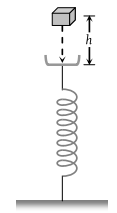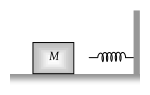An ice cream has a marked value of 700 kcal. How many kilowatt- hour of energy will it deliver to the body as it is digested

(1) 0.81 kWh

(2) 0.90 kWh

(3) 1.11 kWh

(4) 0.71 kWh

Concept Questions :-

Power
High Yielding Test Series + Question Bank - NEET 2020

Difficulty Level:

A running man has half the kinetic energy of that of a boy of half of his mass. The man speeds up by 1m/s so as to have same K.E. as that of the boy. The original speed of the man will be

(1) $\sqrt{2}\text{\hspace{0.17em}}m/s$

(2) $\left(\sqrt{2}-1\right)\text{\hspace{0.17em}}m/s$

(3) $\frac{1}{\left(\sqrt{2}-1\right)}m/s$

(4) $\frac{1}{\sqrt{2}}m/s$

Concept Questions :-

Concept of work
High Yielding Test Series + Question Bank - NEET 2020

Difficulty Level:

A particle of mass m at rest is acted upon by a force F for a time t. Its Kinetic energy after an interval t is

(1) $\frac{{F}^{2}{t}^{2}}{m}$

(2) $\frac{{F}^{2}{t}^{2}}{2m}$

(3) $\frac{{F}^{2}{t}^{2}}{3m}$

(4) $\frac{F\text{\hspace{0.17em}}t}{2m}$

Concept Questions :-

Concept of work
High Yielding Test Series + Question Bank - NEET 2020

Difficulty Level:

Two bodies of masses m and 4 m are moving with equal K.E. The ratio of their linear momentums is

(1) 4 : 1

(2) 1 : 1

(3) 1 : 2

(4) 1 : 4

Concept Questions :-

Concept of work
High Yielding Test Series + Question Bank - NEET 2020

Difficulty Level:

A particle of mass m1 is moving with a velocity v1 and another particle of mass m2 is moving with a velocity v2. Both of them have the same momentum but their different kinetic energies are E1 and E2 respectively. If m1 > m2 then

(1) E1 < E2

(2) $\frac{{E}_{1}}{{E}_{2}}=\frac{{m}_{1}}{{m}_{2}}$

(3) E1 > E2

(4) E1 = E2

Concept Questions :-

Concept of work
High Yielding Test Series + Question Bank - NEET 2020

Difficulty Level:

Four particles are given, having same momentum. Which one has maximum kinetic energy-

(1) Proton

(2) Electron

(3) Deutron

(4) α-particles

Concept Questions :-

Concept of work
High Yielding Test Series + Question Bank - NEET 2020

Difficulty Level:

If a man increase his speed by 2 m/s , his K.E. is doubled, the original speed of the man is

(1) $\left(1+2\sqrt{2}\right)\text{\hspace{0.17em}}m/s$

(2) 4 m/s

(3) $\left(2+2\sqrt{2}\right)\text{\hspace{0.17em}}m/s$

(4) $\left(2+\sqrt{2}\right)\text{\hspace{0.17em}}m/s$

Concept Questions :-

Concept of work
High Yielding Test Series + Question Bank - NEET 2020

Difficulty Level:

A block of mass m initially at rest is dropped from a height h on to a spring of force constant k. the maximum compression in the spring is x then [BCECE 2005](1) $mgh=\frac{1}{2}k{x}^{2}$

(2) $mg\left(h+x\right)=\frac{1}{2}k{x}^{2}$

(3) $mgh=\frac{1}{2}k{\left(x+h\right)}^{2}$

(4) $mg\left(h+x\right)=\frac{1}{2}k{\left(x+h\right)}^{2}$

Concept Questions :-

Elastic potential energy
High Yielding Test Series + Question Bank - NEET 2020

Difficulty Level:

A spherical ball of mass 20 kg is stationary at the top of a hill of height 100 m. It slides down a smooth surface to the ground, then climbs up another hill of height 30 m and finally slides down to a horizontal base at a height of 20 m above the ground. The velocity attained by the ball is

(1) 10 m/s

(2) $10\sqrt{30}$ m/s

(3) 40 m/s

(4) 20 m/s

Concept Questions :-

Gravitational Potential energy
High Yielding Test Series + Question Bank - NEET 2020

Difficulty Level:

The block of mass M moving on the frictionless horizontal surface collides with the spring of spring constant K and compresses it by length L. The maximum momentum of the block after collision is(1) Zero

(2) $\frac{M{L}^{2}}{K}$

(3) $\sqrt{MK}\text{\hspace{0.17em}}L$

(4) $\frac{K{L}^{2}}{2M}$

Concept Questions :-

Elastic potential energy